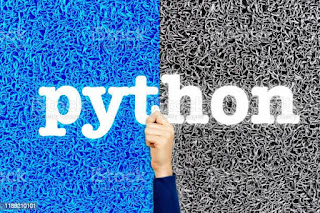## Posts

Showing posts with the label LearnpythonPython

### What is python and its print functionWhat is python and it's print function So let's look at today agenda 1) what is python in easy language 2) how to print hello world in it So hai dear coder welcome to corders land a land of young coders so let start this post so first of all if you know python we'll and good or If you don't know python its excellent so first of if you know python forget about it forget that you know what is python So you forget python right the reason behind it is that if you know some thing your mindset is that so I know python  But the realty is you know a python a little So let's start  So first of all it is a programming language it is used for making web, ai, ml projects So introduction of python is clear that python is a programming language Ok  So let's start with  First statement that is print Yes  Print()  So here we have to type hello world So the method is First of all  You have to write the statement print And then prenthesis()  that men we are telling that computer